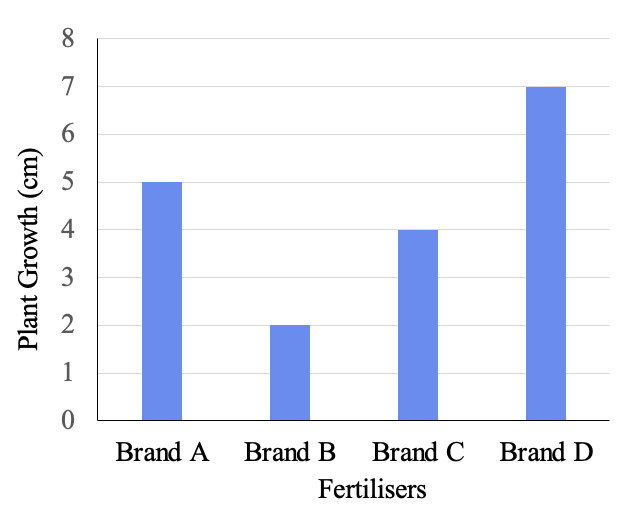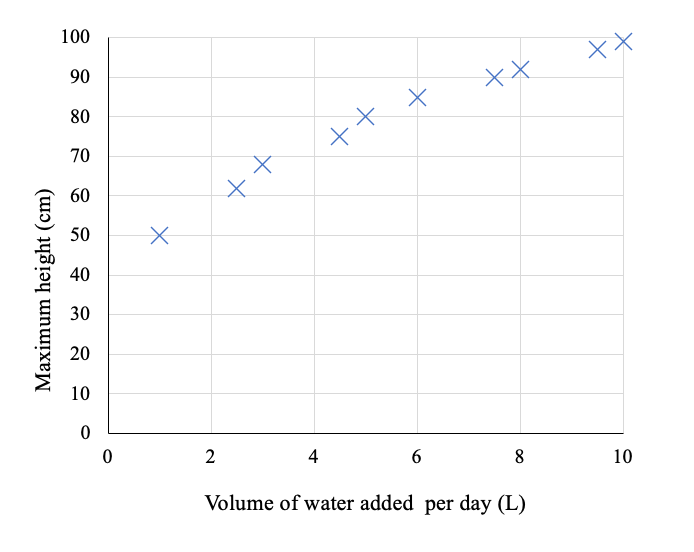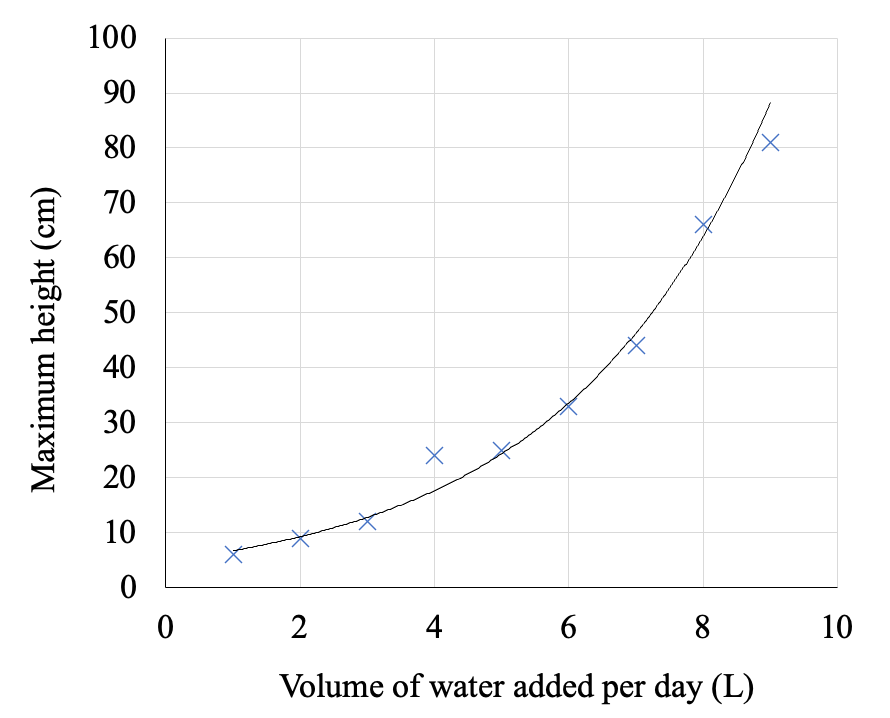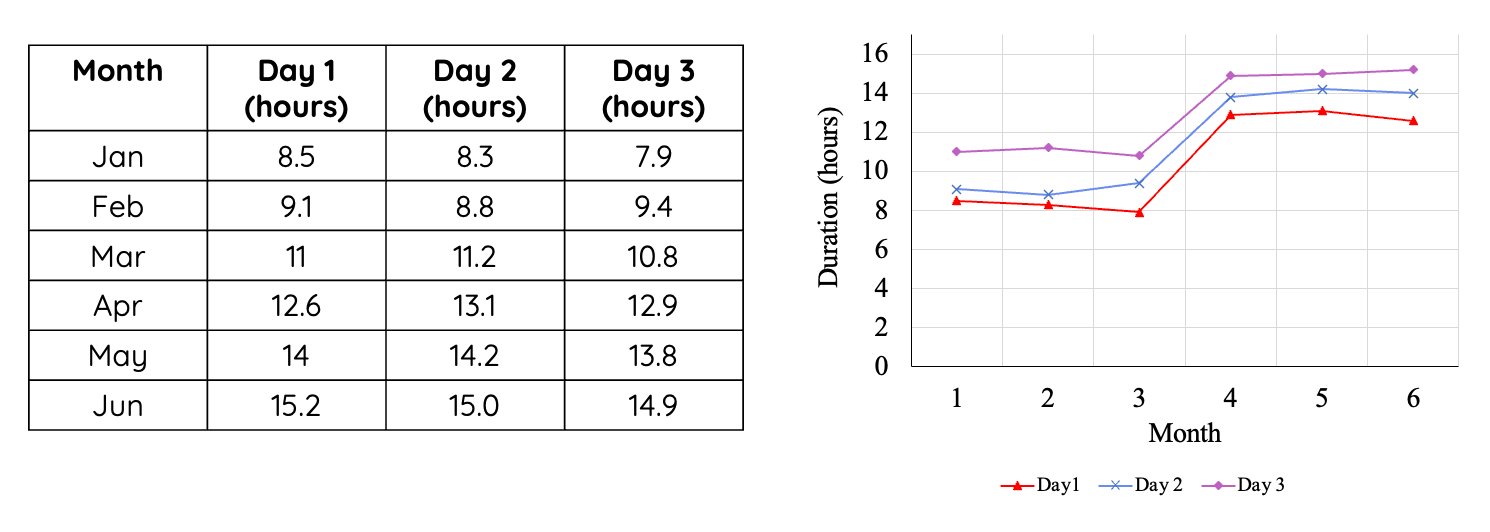# Choosing the Right Type of Graph

This is part of the NSW HSC science curriculum part of the Working Scientifically skills.

### Why do we use graphs?

In science, we often utilise graphs due to their ability to help simplify and visualise complex data sets which make it easier for us to interpret and analyse data. In doing this, they can help us to observe any patterns or trends which might otherwise not have been immediately apparent in the raw data.

There are four types of graphs which you will most likely come across in HSC science:

• Column graph
• Histogram
• Scatter plot
• Line graph

### Column Graphs:

A column graph is a vertical bar graph which displays data using rectangular bars. The height of the bar of the column graph (y-axis) represents magnitude. Each of the individual bars (x-axis) corresponds to a category.

As such, column graphs are ideal for comparing different categories or groups and are used generally when there are a larger number of categories. Another benefit of the column graph is that the categories have a natural hierarchy.

An example of when a column graph may be used is if we were experimenting to see the effects of different brands on fertiliser on plant growth over a period of a month.### Histograms:

A histogram is a column-graph-like chart which uses rectangular bars called "bins" to display data. The height of each bar on the column graph (y-axis) represents population size, while each of the "bins" (x-axis)  represents either a range or a discrete value.

Histograms are utilised to help identify broader patterns in the distribution of data. An example for which we would use a histogram is if we were looking to see how 100 different plants grow over a period of time.### Scatter Plots

Scatter plots present data as individual points n a graph. The scatter plot is used ideally when both the x and the y-axis consists of numerical data. They are used to help identify correlations between two continuous numerical variables where the x-axis represents the independent variables, and the y-axis represents the dependent variable.

Scatter plots are useful because not only can they help to quickly identify relationships between variables, they can also indicate a lack of relationship. Scatter plots would be used for an example where we were investigating the effect of volume of water given each day on plant growth.A line of best fit can be used to help better visualise the trend. Again it is important to keep in mind that the existence of a trend also does not necessarily imply causation. Reasons for correlations to exist may include the fact that there is a third variable which has not been taken into account, or merely coincidence.

A guide on how to draw a line-of-best-fit can be found on the drawing tables and graphs page hereA curve of best fit is like a line-of-best-fit and is used when the trend appears to be non-linear and consists of a single-unbroken line.### Line Graph

A line graph is a type of graph which displays data as a series of data points connected by straight line segments. For line graphs, the x-axis typically represents time while the y-axis can represent any other secondary variable. Thus, they are usually used to identify trends in variables over time.### Summary:

Selecting the right graph depends heavily on the data you are working with and what you want to highlight. It's important to familiarise yourself with different types of graphs and understand when to use each for effective communication of your scientific findings.